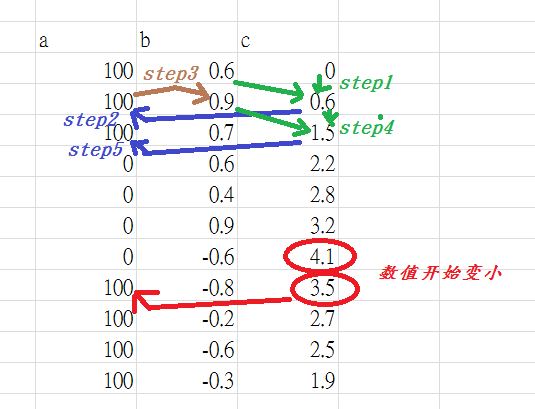2018-11-25 17:43

# matlab同一个地方有2种判断语句c会有2个判断语句

1是当c>2时，a=0

(a只会等于0或100)

``````if c>2

a=0

else

a=100

end
``````

2是当c的值开始递减时,a变成100，并且递减时，不管有没有超过2，a都是100

• 点赞
• 写回答
• 关注问题
• 收藏
• 邀请回答

#### 1条回答默认 最新

•胸毛男 2018-12-06 02:37
``````c=[0,0.6,1.5,2.2,2.8,3.2,4.1,3.5,2.7,2.5,1.9];
iSize = size(c);
a= zeros(1,iSize(1,2));

%超过2过 0没超过 1超过了
k1flag=0;
for i=1:1:iSize(1,2)
if (i==1)
if(c(i)<2)
a(i) = 100;
else
a(i) = 0;
end
else if (~k1flag) %没有超过2过
if(c(i)>2)
k1flag = k1flag+1;
a(i) = 0;
else
a(i) = 100;
end
else %已经超过一次2了
if (c(i)>=c(i-1)) %超过2上升中
a(i)=0;
else if(c(i)<c(i-1)) %超过2下降中
a(i)=100;
end
end
end
end
end
``````
点赞 评论